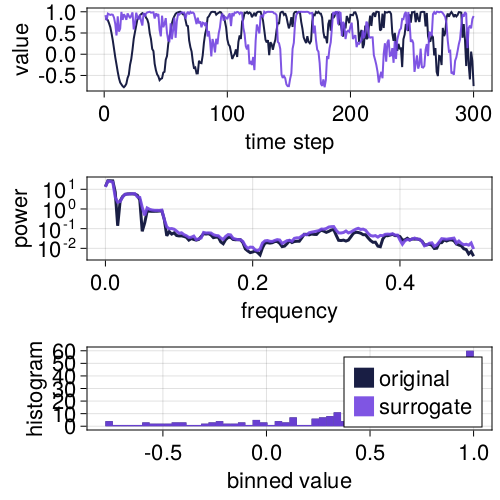# Crash-course in timeseries surrogate testing

Note

The summary here follows Sect. 7.4 from Nonlinear Dynamics by Datseris and Parlitz.

## What is a surrogate timeseries?

A surrogate of a timeseries x is another timeseries s of equal length to x. This surrogate s is generated from x so that it roughly preserves one or many pre-defined properties of x, but is otherwise randomized.

The upper panel in the figure below shows an example of a timeseries and one surrogate realization that preserves its both power spectrum and its amplitude distribution (histogram). Because of this preservation, the time series look similar.

using TimeseriesSurrogates, CairoMakie
x = LinRange(0, 20π, 300) .+ 0.05 .* rand(300)
ts = sin.(x./rand(20:30, 300) + cos.(x))
s = surrogate(ts, IAAFT())

surroplot(ts, s)## Performing surrogate hypothesis tests

A surrogate test is a statistical test of whether a given timeseries satisfies or not a given hypothesis regarding its properties or origin.

For example, the first surrogate methods were created to test the hypothesis, whether a given timeseries x that appears noisy may be the result of a linear stochastic process or not. If not, it may be a nonlinear process contaminated with observational noise. For the suitable hypothesis to test for, see the documentation strings of provided Surrogate methods or, even better, the review from Lancaster et al. (2018)[Lancaster2018].

To perform such a surrogate test, you need to:

1. Decide what hypothesis to test against
2. Pick a surrogate generating method that satisfies the chosen hypothesis
3. Pick a suitable discriminatory statistic q with q(x) ∈ Real. It must be a statistic that would obtain sufficiently different values for timeseries satisfying, or not, the chosen hypothesis.
4. Compute q(s) for thousands of surrogate realizations s = surrogate(x, method)
5. Compare q(x) with the distribution of q(s). If q(x) is significantly outside the e.g., 5-95 confidence interval of the distribution, the hypothesis is rejected.

This whole process is automated by SurrogateTest, see the example below.

## An educative example

Let's put everything together now to showcase how one would use this package to e.g., distinguish deterministic chaos contaminated with noise from actual stochastic timeseries, using the permutation entropy as a discriminatory statistic.

First, let's visualize the timeseries

using TimeseriesSurrogates # for surrogate tests
using DynamicalSystemsBase # to simulate logistic map
using ComplexityMeasures   # to compute permutation entropy
using Random: Xoshiro      # for reproducibility
using CairoMakie           # for plotting

# AR1
n = 400 # timeseries length
rng = Xoshiro(1234567)
x = TimeseriesSurrogates.AR1(; n_steps = n, k = 0.25, rng)
# Logistic
logistic_rule(x, p, n) = @inbounds SVector(p*x*(1 - x))
ds = DeterministicIteratedMap(logistic_rule, [0.4], [4.0])
Y, t = trajectory(ds, n-1)
y = standardize(Y[:, 1]) .+ 0.5randn(rng, n) # 50% observational noise
# Plot
fig, ax1 = lines(y)
ax2, = lines(fig[2,1], x, color = Cycled(2))
ax1.title = "deterministic + 50%noise"
ax2.title = "stochastic AR1"
fig

Then, let's compute surrogate distributions for both timeseries using the permutation entropy as the discriminatory statistic and RandomFourier as the surrogate generation method

perment(x) = entropy_normalized(SymbolicPermutation(; m = 3), x)
method = RandomFourier()

fig = Figure()
axs = [Axis(fig[1, i]) for i in 1:2]
Nsurr = 1000

for (i, z) in enumerate((y, x))
sgen = surrogenerator(z, method)
qx = perment(z)
qs = map(perment, (sgen() for _ in 1:Nsurr))
hist!(axs[i], qs; label = "pdf of q(s)", color = Cycled(i))
vlines!(axs[i], qx; linewidth = 5, label = "q(x)", color = Cycled(3))
axislegend(axs[i])
end

fig

we clearly see that the discriminatory value for the deterministic signal is so far out of the distribution that the null hypothesis that the timeseries is stochastic can be discarded with ease.

This whole process can be easily automated with SurrogateTest as follows:

test = SurrogateTest(perment, y, method; n = 1000, rng)
p = pvalue(test)
p < 0.001  # 99.9-th quantile confidence
• Lancaster2018Lancaster, G., Iatsenko, D., Pidde, A., Ticcinelli, V., & Stefanovska, A. (2018). Surrogate data for hypothesis testing of physical systems. Physics Reports, 748, 1–60. doi:10.1016/j.physrep.2018.06.001